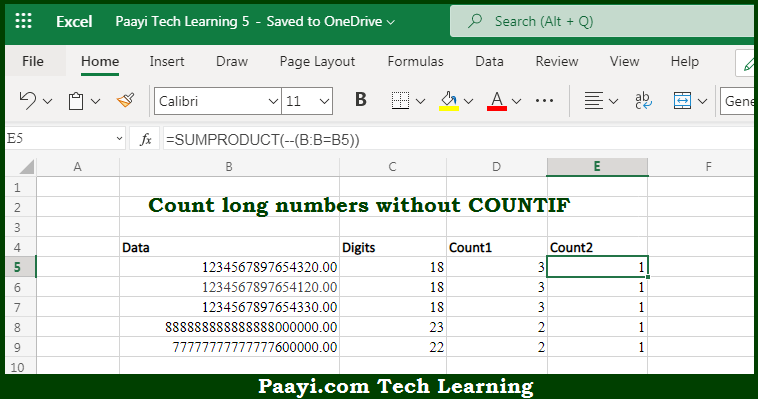# Learn How to Count Long Numbers Without COUNTIF in Microsoft Excel

Written by | 0 Comments | 426 Views

In this article, you will learn how to COUNT various things in Microsoft Excel using a single or combination of functions and its purpose in Microsoft Excel. You will also get to know how to Count Long Numbers Without COUNTIF and see the generic formula.

Count Long Numbers Without COUNTIF in Microsoft Excel

The main purpose of this formula is to count the long numbers without using the COUNTIF function. Here we will learn how to count the long numbers in the given cell without using COUNTIF in Microsoft Excel. That implies, with the help of a formula based on the SUMPRODUCT function you can able to count long numbers without using the COUNTIF function. The reason here for using the SUMPRODUCT function is that it is more efficient and smooth and COUNTIF. And, there are chances that COUNIF may give wrong results. So, with the help of this formula, you can able to count the long numbers without using the COUNTIF function.

General Formula to Count Long Numbers Without COUNTIF

SUMPRODUCT(--(A:A=A1))

The Explanation for Count Long Numbers Without COUNTIFSo we know that with the help of the given formula above you can able to count the long numbers without using the COUNTIF function. Here we will learn how to count the long numbers in the given cell without using COUNTIF in Microsoft Excel. As we know that, the expression inside the SUMPRODUCT function compares all the values in the named range "data" with the value from the given column in the current row. Resulting in an array of TRUE/FALSE results. After that, the double negative coerces the TRUE/FALSE values to 1/0 values. And at last, the SUMPRODUCT function simply sums the items in the array and returns the result. So, with the help of this formula, you can able to count the long numbers without using the COUNTIF function.